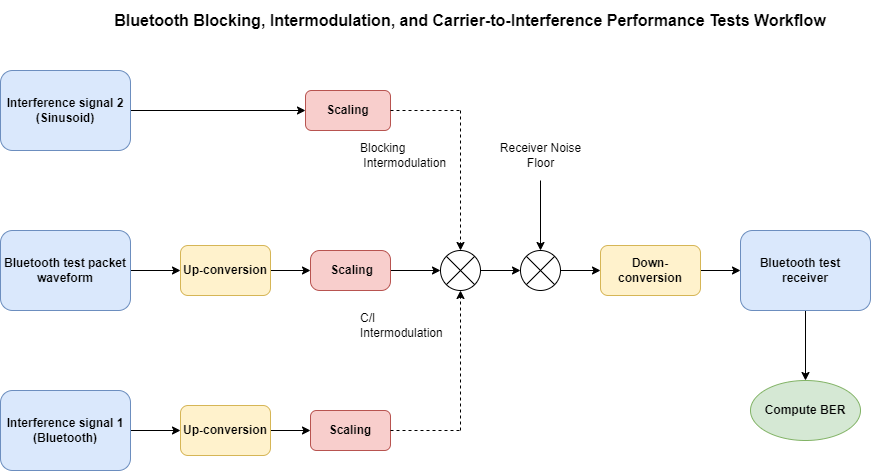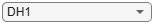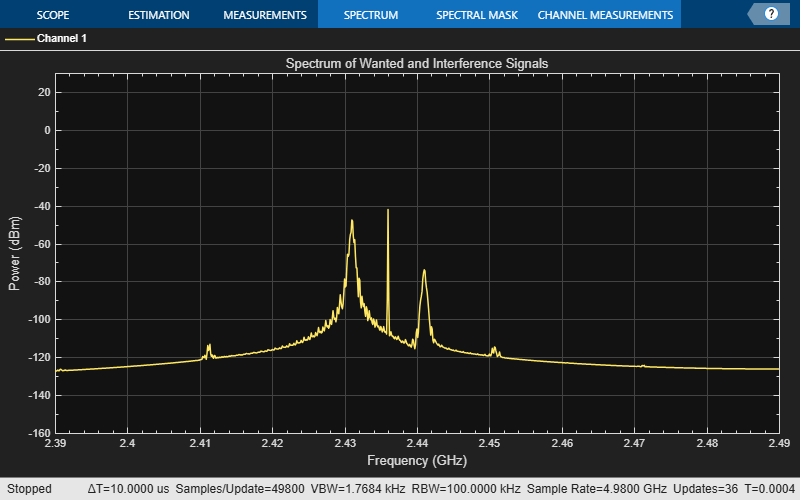# Bluetooth BR/EDR Blocking, Intermodulation, and Carrier-to-Interference Performance Tests

This example shows how to perform Bluetooth® basic rate/enhanced data rate (BR/EDR) radio frequency (RF) receiver tests specific to blocking, intermodulation, and carrier-to-interference (C/I) according to the Bluetooth RF Test Specifications . The example also verifies whether these test measurement values are within the limits specified by the Bluetooth RF Test Specifications .

### Objectives of Bluetooth RF tests

The Bluetooth RF Test Specifications  defined by the Bluetooth Special Interest Group (SIG) includes RF tests for both transmitters and receivers. The objectives of these RF tests are to:

• Ensure interoperability between all Bluetooth devices.

• Ensure a basic level of system performance for all Bluetooth products.

Each test case has a specified test procedure and an expected outcome, that must be met by the implementation under test (IUT).

The Bluetooth receiver tests are designed to ensure that the IUT can receive data over a range of conditions. The transmitted signal has high power, and the receiver can achieve a defined bit error rate (BER) in the presence of in-band and out-of-band interference. This example includes three Bluetooth BR and EDR RF receiver tests specific to blocking, intermodulation, and C/I performance according to the Bluetooth RF Test Specifications .

• Blocking Performance - The blocking performance test verifies the receiver performance in the presence of out-of-band interfering signals, operating outside the 2402 MHz - 2480 MHz band. This test only applies for BR mode.

• Intermodulation Performance - The intermodulation performance test verifies the receiver performance in the presence of unwanted signals near in frequency. This test only applies for BR mode.

• C/I Performance - The C/I performance test verifies the receiver performance in the presence of adjacent and co-channel interfering signals.

Because the wanted signal is not the only signal transmitting in the given frequency range, these RF tests are essential to verify the receiver performance.

This block diagram summarizes the example flow.To simulate the interference tests, perform these steps.

1. Generate test packets and use the function `bluetoothTestWaveform` to generate a Bluetooth BR test waveform.

2. Perform frequency up conversion to obtain a passband signal.

3. Scale the transmitted signal to a desired input level.

4. Add the interference signal(s) depending on the performance test.

6. At the receiver, down convert the signal and then demodulate, decode, and calculate BER on the payload.

7. Compare the obtained BER with the reference BER and detect any spurious frequencies.

### Initialize the Simulation Parameters

Specify the RF receiver performance test, physical layer (PHY) transmission mode and packet type you want to simulate.

```rxPerformanceTest ="Intermodulation"; phyMode ="BR"; packetType ="DH1"; if rxPerformanceTest ~= "C/I" && phyMode ~= "BR" error("For EDR modes, only C/I performance test is specified.") end```

Specify the operating frequency for the IUT based on the performance test.

```% ---------------------------------------------------- % Operating | | % Frequency | C/I | Blocking | Intermodulation | % (MHz) | | % ---------------------------------------------------- % Low | 2405 | - | 2402 | % Mid | 2441 | 2460 | 2441 | % High | 2477 | - | 2480 | % ----------------------------------------------------- Fc = 2441e6; % Operating frequency in Hz```

Specify the symbol rate, number of samples per symbol, and sampling rate.

```Rsym = 1e6; % Symbol rate in symbols per second sps = 20; % Number of samples per symbol Fs = Rsym*sps; % Sampling rate in samples per second```

### Generate Baseband Waveforms

To generate a wanted signal with a PRBS9 payload and DH1 packet type, create a Bluetooth test waveform configuration object.

```wantedTestWaveformConfig = bluetoothTestWaveformConfig; wantedTestWaveformConfig.Mode = phyMode; wantedTestWaveformConfig.PacketType = packetType; wantedTestWaveformConfig.PayloadType = 0; % Payload type for PRBS9 sequence if phyMode ~= "BR" wantedTestWaveformConfig.WhitenStatus = "On"; end wantedTestWaveformConfig.SamplesPerSymbol = sps;```

Generate a wanted signal from the specified configuration.

`wantedWaveform = bluetoothTestWaveform(wantedTestWaveformConfig);`

To generate an interference signal with a PRBS15 payload and BR PHY mode, create a Bluetooth test waveform configuration object.

```interferenceTestWaveformConfig = bluetoothTestWaveformConfig; interferenceTestWaveformConfig.Mode = "BR"; interferenceTestWaveformConfig.PacketType = "DH1"; interferenceTestWaveformConfig.PayloadType = 3; % Payload type for PRBS15 sequence interferenceTestWaveformConfig.SamplesPerSymbol = sps;```

Generate an interference signal from the specified configuration.

`interferenceWaveform1 = bluetoothTestWaveform(interferenceTestWaveformConfig);`

To generate an interference signal with a PRBS15 payload and PHY transmission mode same as that of wanted signal, create a Bluetooth test waveform configuration object.

```interferenceTestWaveformConfig = bluetoothTestWaveformConfig; interferenceTestWaveformConfig.Mode = phyMode; interferenceTestWaveformConfig.PacketType = packetType; interferenceTestWaveformConfig.PayloadType = 3; % Payload type for PRBS15 sequence interferenceTestWaveformConfig.SamplesPerSymbol = sps; interferenceWaveform11 = bluetoothTestWaveform(interferenceTestWaveformConfig);```

### Frequency Up-conversion

Create a digital up-converter System object™ to perform frequency up-conversion.

```% Interpolation factor for up-conversion to cover Bluetooth RF frequency band interpFactor = ceil(2*2490e6/Fs); upConv = dsp.DigitalUpConverter( ... InterpolationFactor=interpFactor,... SampleRate=Fs,... Bandwidth=Rsym,... StopbandAttenuation=44,... PassbandRipple=0.5,... CenterFrequency=Fc);```

Up-convert the baseband waveform to passband.

```wantedWaveformUp = upConv(wantedWaveform); release(upConv);```

### Generate Test Parameters

Generate test parameters based on the type of performance test and the operating frequency. Generate the interference frequencies and corresponding scaling factors (alpha, beta, and gamma) by using the helperBluetoothTestParamGenerate helper function.

```[alpha,beta,gamma,interferenceFreq1,interferenceFreq2] = ... helperBluetoothTestParamGenerate(rxPerformanceTest,Fc,phyMode); pktCnt = 10; % Number of packets totInterferenceParams = length(interferenceFreq1); % Total interfering frequencies simInterferenceParams = min(totInterferenceParams,6); % Interfering ferequencies used in simulation % Time vector to generate sinusoidal unmodulated interference signal, % interference signal #2. t = (0:(length(wantedWaveformUp)-1)).'/(interpFactor*Fs);```

### Simulate RF Receiver Performance Tests

The example simulates the Bluetooth RF performance tests as follows:

• For Blocking performance, there is only one interference signal, interference signal #2. So, the scaling factor (beta) for interference signal #1 is 0.

• For Intermodulation performance, there are two interference signals.

• For C/I performance, there is only one interference signal, interference signal #1. So, the scaling factor (gamma) for interference signal #2 is 0.

Create a digital down-converter System object™ to perform frequency down-conversion.

```downConv = dsp.DigitalDownConverter(... DecimationFactor=interpFactor,... SampleRate=Fs*interpFactor,... Bandwidth=Rsym,... StopbandAttenuation=44,... PassbandRipple=0.5,... CenterFrequency=Fc);```

Create an automatic gain control System object™.

`agc = comm.AGC(DesiredOutputPower=1);`

Create a thermal noise System object™.

```NF = 12; % Noise figure (dB) thNoise = comm.ThermalNoise(NoiseMethod="Noise figure",... SampleRate=interpFactor*Fs,... NoiseFigure=NF);```

```rxConfig = bluetoothPhyConfig; rxConfig.Mode = phyMode; if phyMode == "BR" rxConfig.WhitenStatus = "Off"; end if contains(packetType,"EV") rxConfig.HasExtendedSCO = true; rxConfig.ExtendedSCOPayloadLength = wantedTestWaveformConfig.FixedPayloadLength; end rxConfig.SamplesPerSymbol = sps;```

Generate payload bits to calculate BER.

```payload = bluetoothTestData(wantedTestWaveformConfig.FixedPayloadLength); payloadLen = wantedTestWaveformConfig.FixedPayloadLength; % In bytes```

Create and configure a spectrum analyzer to visualize the spectrum of wanted and interference signals.

```spectrumScope = spectrumAnalyzer(... SampleRate=Fs*interpFactor,... SpectralAverages=10,... YLimits=[-160 30], ... Title="Spectrum of Wanted and Interference Signals",... SpectrumUnits="dBm",... ShowLegend=true,... FrequencySpan="Start and stop frequencies",... StartFrequency=2390e6,... StopFrequency=2490e6,... RBWSource="Property",... RBW=1e5);```

Based on the RF receiver test you choose, up-converter creates interfering signals on different channels in every for loop iteration. The BER is computed on the wanted channel.

```numSpuriousFreq = 0; % Initialize counter for f=1:simInterferenceParams % Upconvert interference waveform #1 upConv.CenterFrequency = interferenceFreq1(f); if phyMode == "BR" interferenceWaveform1Up = upConv(interferenceWaveform1); else if rxPerformanceTest == "C/I" if f == 1 % co-channel interferenceWaveform1Up = upConv(interferenceWaveform11); else interferenceWaveform1Up = upConv([interferenceWaveform1;zeros(length(wantedWaveform)-length(interferenceWaveform1),1)]); end else interferenceWaveform1Up = upConv(interferenceWaveform11); end end % Generate an interference waveform #2 which is a sinusoidal % unmodulated signal. The sqrt(2) factor ensures that the power of the % sinusoidal signal is normalized. interferenceWaveform2 = sqrt(2)*sin(2*pi*interferenceFreq2(f)*t); % Add the interference signals to wanted signal rxWaveform = alpha(f)*wantedWaveformUp+beta(f)*interferenceWaveform1Up+gamma(f)*interferenceWaveform2; % Plot the spectrum spectrumScope(rxWaveform) bitsErr = 0; % Initialize variable for n = 1:pktCnt chanOut = thNoise(complex(rxWaveform)); % Add thermal noise to the signal downConvOut = downConv(real(chanOut)); % Perform frequency down conversion agcOut = agc(downConvOut); % Apply AGC rxIn = [agcOut;complex(zeros(25000-length(agcOut),1))]; % Append zeros at the end to match the input expected size [decBits,decodedInfo,pktValid] = bluetoothIdealReceiver(rxIn,rxConfig); % Packets with header failure are not considered in BER calculation if pktValid && decodedInfo.PayloadLength == payloadLen bitsErr = bitsErr + sum(decBits~=payload); end end % Determine BER ber = bitsErr/(pktCnt*8*payloadLen); if rxPerformanceTest=="Intermodulation" fprintf("Measured BER for interfering signals at %f Hz and %f Hz is %f.\n", interferenceFreq1(f), interferenceFreq2(f), ber); else fprintf("Measured BER for interfering signal at %f Hz is %f.\n", interferenceFreq1(f), ber); end % Compute the number of spurious frequencies detected if ber > 1e-3 numSpuriousFreq = numSpuriousFreq + 1; fprintf("Spurious frequency detected !!") end release(upConv); release(downConv); release(agc); release(spectrumScope); end```
```Measured BER for interfering signals at 2447000000.000000 Hz and 2444000000.000000 Hz is 0.000000. Measured BER for interfering signals at 2449000000.000000 Hz and 2445000000.000000 Hz is 0.000000. Measured BER for interfering signals at 2451000000.000000 Hz and 2446000000.000000 Hz is 0.000000. Measured BER for interfering signals at 2435000000.000000 Hz and 2438000000.000000 Hz is 0.000000. Measured BER for interfering signals at 2433000000.000000 Hz and 2437000000.000000 Hz is 0.000000. Measured BER for interfering signals at 2431000000.000000 Hz and 2436000000.000000 Hz is 0.000000. ```### Reference Results

Display the test results. The test verdict depends on the number of spurious frequencies detected.

```testPass = false; if rxPerformanceTest == "C/I" && numSpuriousFreq <= 5 testPass = true; elseif rxPerformanceTest == "Blocking" && numSpuriousFreq <= 24 testPass = true; elseif rxPerformanceTest == "Intermodulation" && numSpuriousFreq == 0 testPass = true; end if testPass fprintf("%s performance test passed.\n",rxPerformanceTest); else fprintf("%s performance test failed.\n",rxPerformanceTest); end```
```Intermodulation performance test passed. ```

### Appendix

The example uses these helper functions:

### Selected Bibliography

1. Bluetooth Special Interest Group (SIG). “Bluetooth RF Test Specification”, RF.TS.p32 Section 4.5. 2022. https://www.bluetooth.com.

2. Bluetooth Special Interest Group (SIG). "Core System Package [BR/EDR Controller Volume]". Bluetooth Core Specification. Version 5.3, Volume 2. https://www.bluetooth.com.

### Local Functions

```function payload = bluetoothTestData(payloadLen) % Generate the test packet payload, a PRBS9 sequence based on payload length polynomial = "z^9+z5+1"; initialConditions = ones(1,9); pnSeq = comm.PNSequence(Polynomial=polynomial, ... InitialConditions=initialConditions, SamplesPerFrame=payloadLen*8); payload = pnSeq(); end```